• # Calc AB ReviewRemember: Derivative of log_a(u) = du/(xlna)
Derivative of arcsec(u) = du/(abs(u)(u^2-1)^.5)
Integrals of Inverse Trig: arcsin -> has no 1/a.
arcsec-> has abs value around u.
• Period: to

• # Diff EQs and Logistics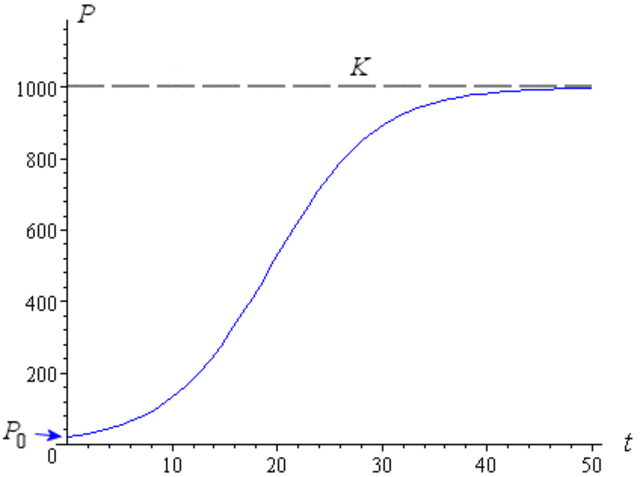Remember: When presented with logistic in the form of dP/dt=kP((M-P), the resulting equation is M/(1+Ae^(-kMt)). If presented with dP/dt=kP(1-P/M), the resulting equation is M/(1+Ae^(-kt))
• # AP Practice Problems 1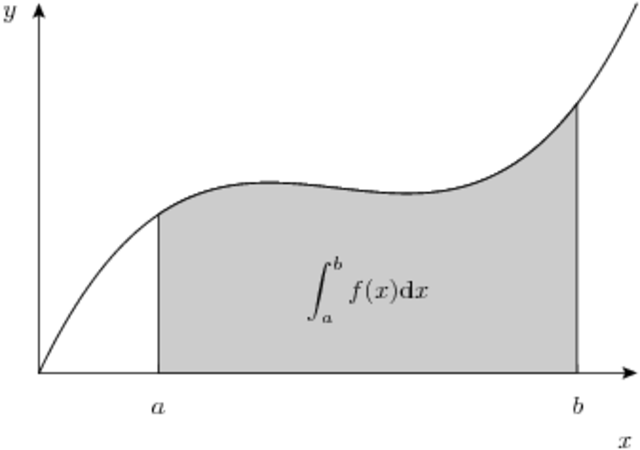When sketching integrals, always look for key points on derivative graph: Zeroes, which imply extrema, and extrema, which are PPOIs (Possible Points of Inflection).
• # Practice AP Problems 2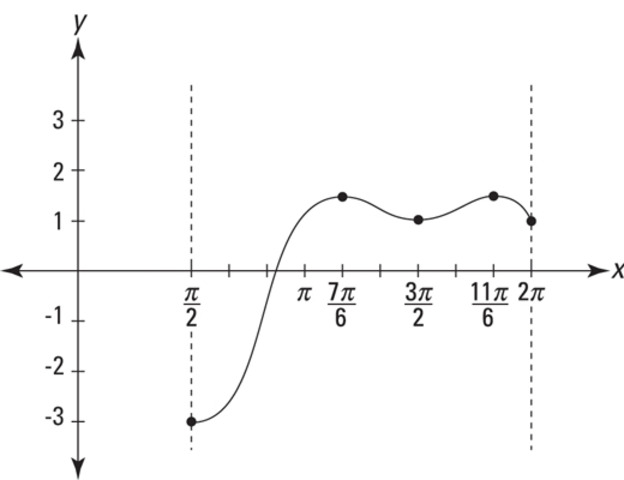Remember: When given a function whose derivative doesn't experience a sign change over the given domain, the endpoints are absolute extrema, not relative extrema.
• # Self Review: Volumes of Solids

Remember that when using the disc method, multiply the integral by pi, and when using the shell method, multiply the integral by 2pi.

Shell: (length)(distance from line of rotation)
Disc: (equation)^2-(other equation if it exists)^2 (also known as washer method).
• # Complicated Integrals and Practice AP Problems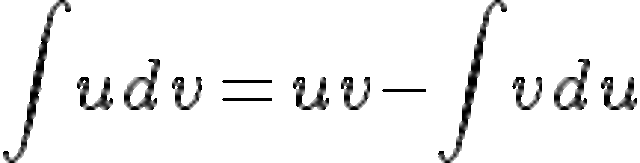Remember LIPET for parts (Logs, Inverse-trig, Polynomials, Exponentials, Trig) Try to massasge functions to look like something that's easily integratable. Adding and subtracting to the numerator is generally a very effective method. To find average speed of a particle given by parametrics, find the arclength, and multiply it by 1/(b-a).
• # Review of Practice AP Exam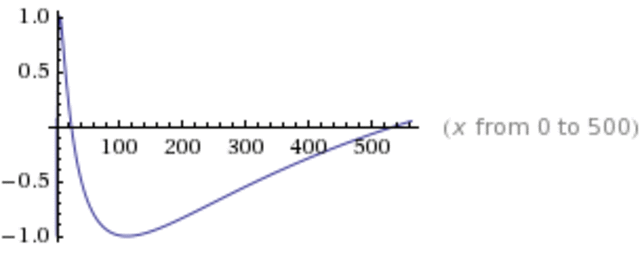Do not always trust your calculator when finding zeroes; think about the actual equation. Pi/3 is absolutely not less than one. Try to recognize the limit definition of the integral when you see it. Usually characterized by a series and 1/n.
• # Geometric Series Review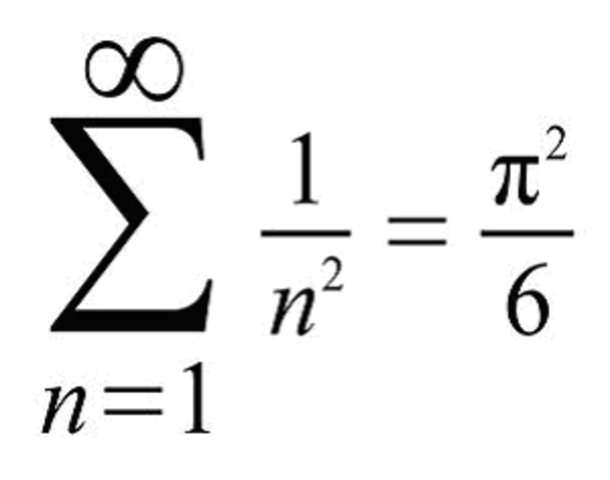Remember that when finding the sum of a geometric series, the formula for convergence is a/(1-r). If the geometric series contains a variable, find when r < 1.

If you're trying to convert a convergence formula into a geometric series, you must massage the equation a bit to make it look like a/(1-r) i.e. divide until the constant is equal to 1, and until the "r" is negative.
• # Taylor Polynomial/Series ReviewDefinition of Taylor Polynomial: f(c)+f '(c)(x-c)+f ''(c)(x-c)^2/2!+... + f^n(c)(x-c)^n/n!. A Maclaurian polynomial is just a Taylor polynomial centered at zero.
To integrate/differentiate Taylor Polynomials/Series, simply proceed as you would with any other equation.
If you're given a problem that asks for a taylor polynomial centered about pi/2 of sinx or cosx, the resultant polynomial will be equal to cosx or sinx respectively.
• # Polar Review (Personal day, I was absent)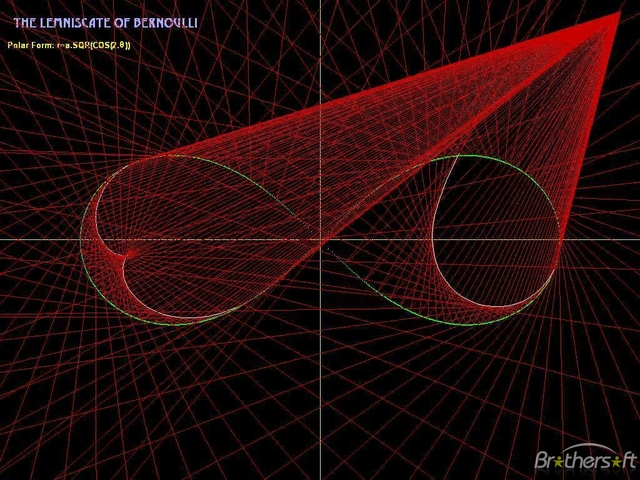Remember that when finding the area of a region bounded by a polar curve, the equation is equal to
1/2 int(theta1)_(theta2) (curve)^2 dtheta. If the area is bounded by two curves, find the "shaded" area of the first curve and add the remaining area of the second curve. When converting to parametric: x=rcos(-) y=rsin(-)
• # Personal ReflectionsI'm feeling very confident at the moment.
I just need to remember that there is no longer a penalty for wrong answers. Guess if you don't have time/you don't know the answer. Also, if you must guess, stick with one letter throughout the entirety of the test; statistically, you'll have a better chance of getting it right.
• # THE BIG GAMEOverall I thought the test was very easy. I had trouble with problem 6 on the free response, and probably should have studied Taylor Polynomials a bit more, but other than that, it was quite straightfoward.
Another important thing to note is that there are no longer three calculator questions. There are only two, with the remaining for being non-calculator.